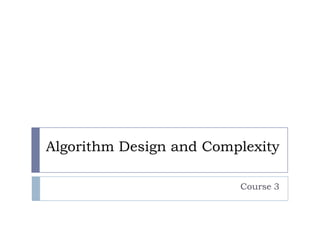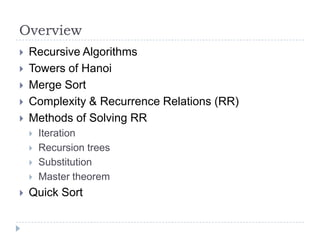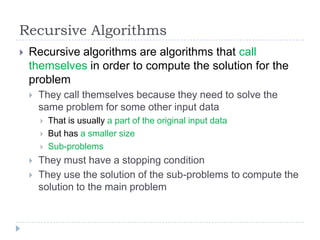Successfully reported this slideshow.

# Algorithm Design and Complexity - Course 3

21

Share×
1 of 49
1 of 49

# Algorithm Design and Complexity - Course 3

21

Share

## More Related Content

### Related Books

Free with a 14 day trial from Scribd

See all

### Related Audiobooks

Free with a 14 day trial from Scribd

See all

### Algorithm Design and Complexity - Course 3

1. 1. Algorithm Design and Complexity Course 3
2. 2. Overview      Recursive Algorithms Towers of Hanoi Merge Sort Complexity & Recurrence Relations (RR) Methods of Solving RR      Iteration Recursion trees Substitution Master theorem Quick Sort
3. 3. Recursive Algorithms  Recursive algorithms are algorithms that call themselves in order to compute the solution for the problem  They call themselves because they need to solve the same problem for some other input data      That is usually a part of the original input data But has a smaller size Sub-problems They must have a stopping condition They use the solution of the sub-problems to compute the solution to the main problem
4. 4. Recursive Algorithms (2)   Generic recursive algorithm It is difficult to find a generic formula to recursive algorithms Compute_Recursive(A[1..n]) IF (n <= 1) // end of recursion RETURN SIMPLE_A_SOL Preprocess(A) B[1..k] = Divide(A) // smaller subproblems FOR (i = 1.. k) B_SOL[i] = Compute_Recursive(B[i][1..ni]) // recursion A_SOL = Combine(B[1..k]) // combine solutions RETURN A_SOL
5. 5. Complexity of Recursive Algorithms  Because the algorithms/procedures call themselves:    The running time shall be described by a recursive equation T(n) = Time_for(iterative part, n) + Time_for(recurse part, n) T(n) is the running time for Compute_Recursive Time_for means the running time for parts of the algorithm: it depends on the size of the input data for the current call of Compute_Recursive  they depend of n
6. 6. Recurrence Relations  T(n) = Time_for(Preprocess, n) Time_for(Divide, n) Time_for(Combine, n)) T(n1) … T(nk) where n1 , … nk < n + + + +
7. 7. Design Technique: Divide et Impera   Step 1: Divide the problem into simpler subproblems of smaller size Step 2: Solve the sub-problems using the same method as for solving the main problem (Impera)  If the sub-problems are simple enough, solve them iteratively  Step 3: Combine the solutions to the sub-problems in order to find the solutions to the main problem  Technique adapted to algorithms from politics, war, etc (as old as the Roman Empire)
8. 8. Design Technique: Divide et Impera (2)  There are some general recurrence relations for D&I  T(n) = a * T(n / b) + C(n) + D(n)    b > 1  number of sub-problems of equal size 1 <= a ( <= b usually)  number of sub-problems that are solved T(n) = a * T (n - b) + C(n) + D(n)  Decrease et impera
9. 9. Towers of Hanoi     http://en.wikipedia.org/wiki/Tower_of_Hanoi Given three rods and a number of disks of different sizes which can slide onto any rod. All the disks are placed in a neat stack in ascending order of size on one rod, the smallest at the top, thus making a conical shape. The objective of the puzzle is to move the entire stack to another rod, obeying the following rules:    Only one disk may be moved at a time. Each move consists of taking the upper disk from one of the rods and sliding it onto another rod, on top of the other disks that may already be present on that rod. No disk may be placed on top of a smaller disk.
10. 10. Towers of Hanoi (2)
11. 11. Towers of Hanoi – Recursive Algorithm  Procedure for recursive solution:     label the pegs A, B, C—these labels may move at different steps let n be the total number of discs number the discs from 1 (smallest, topmost) to n (largest, bottommost) To move n discs from peg A to peg C:    move n−1 discs from A to B. This leaves disc #n alone on peg A move disc #n from A to C move n−1 discs from B to C so they sit on disc #n
12. 12. Towers of Hanoi–Recursive Algorithm (2) Hanoi(N, Src, Aux, Dst) IF (N <= 1 ) RETURN Hanoi(N - 1, Src, Dst, Aux) Move from Src to Dst Hanoi(N - 1, Aux, Src, Dst) Iterative complexity: (1) Recursive complexity: 2 * T(N - 1) T(N) = 2 * T(N - 1) + (1) Complexity??
13. 13. Legend    Indian temple with 64 disks The priests move the disks according to the rules of the problem The world will end when they will finish arranging the disks on the new pillar T(N) T(N - 1) … T(2) T(1) = 2 * T(N - 1) + = 2 * T(N - 2) + T(N) = = 2 * T(1) + = (1) (1) (1) (1) |*2 | * 2N-2 | * 2N-1 (1) * (1 + 2 + … + 2N-1) = 2N * (1) = (2N)
14. 14. Conclusion    If 1 second needed to move a disk from a pillar to another 264 seconds are needed to complete the task Almost 16 * 1018 seconds
15. 15. Merge Sort MergeSort(A, p, r) IF (p >= r) // stop condition; n = r – p <= 0 RETURN; q = floor((p+r) / 2) // split the array in two // (equal) halves MergeSort(A, p, q) // A1 - sorted MergeSort(A, q+1, r) // A2 - sorted Merge(A, p, q, r) // merge 2 sorted sub// arrays of half-size RETURN; Initial call: MergeSort(A, 1, n)
16. 16. Merge Sort – Running Time  T(n) = Recursive Running Time + Iterative Running Time Recursive RT = 2 * T(n / 2) Iterative RT = (n) + (1) = (n)  Thus:   T(n) = 2 * T(n / 2) +  (n) In order to compute the order of growth of recursive algorithms, we need to know how to compute the solution to recurrence relations!
17. 17. Recurrence Relations  T(n) = T(n-1) + 1 T(n) = T(n-k) + n T(n) = T(n/2) + 1 T(n) = 5*T(n/3) + n log n T(n) = T(sqrt(n)) + 1 … Stop condition: usually T(1) =  Can be solved using 4 methods:           Iteration Recursion Trees Substitution Master Theorem (1)
18. 18. Recurrence Relations (2)
19. 19. Technical Issues - Simplifications     Use of floors and ceilings Compute exact vs. asymptotic solution for a recurrence relation Stopping / boundary conditions The iterative and recursion trees methods are not strong mathematical methods for using recurrences   The substitution method and Master theorem are strong mathematical methods as they use:    Because they use partial induction Complete induction: substitution method A proven theorem: Master method However, I accept any method as long as the result is correct!
20. 20. Iteration Method     Algebraic method Simplest way to solve recurrences May be very difficult to solve some of them Expand the terms that are on the right side of the recurrence, by using the same formula and the new parameter (size of the input data) T(n) T(n - 1) … T(n - k) … T(2) = T(n-n+2) T(1) Add them up: T(n) = T(n - 1) + n = T(n - 2) + n - 1 = T(n - k - 1) + n - k = T(1) + 2 =1 =1+2+…+n= (n2)
21. 21. Iteration Method (2)  Advantages:    Very simple Can compute exact and asymptotical solutions Disadvantages:   Not mathematically rigorous Difficult to solve some recurrences T(n) = T(2n/3) + T(n/3) + n T(n) = T(n/4) + T(n/2) + 1
22. 22. Iteration Method – Exercises  T(n) = 2 * T(n/2) +  (n) Solved on whiteboard  T(n) = T(sqrt(n)) + 1 T(n) = T(sqrt(n)) + n  Sometimes, it is helpful to use substitutions:    Of variables (e.g. let n = 2k) Of recurrences (e.g. on whiteboard)
23. 23. Recursion Trees  Similar to the iteration method Uses a graphical model for expanding the recurrent terms that are not known The result is a recursion tree  E.g. T(n) = T(n-1) + n  
24. 24. Recursion Trees (2)      For each recurrent term on the right side of the recurrence relation, expand it into a child of the current node Continue this process recursively, until stopping conditions are reached The complexity of a node is the sum of the complexities in the nodes that are part of the entire sub-tree dominated by that node => The solution of the recurrence relation is the complexity of the root node => The solution of the recurrence relation is the sum of the complexities of each node in the tree  For simplifying the procedure, sum up on each level of the tree in order to notice a possible pattern => incomplete induction
25. 25. Recursion Trees – Exercises  T(n) = 2 * T(n/2) +   (n) Solved on whiteboard T(n) = T(n/3) + T(2n/3) + (n) => T(n) = (n logn)
26. 26. Recursion Trees – Exercises (2)  T(n) = T(n/2) + T(n/4) + T(n/8) + n
27. 27. Iteration Method – Exercises (3)  T(n) = T(n/2) + T(n/4) + T(n/8) + n => T(n) = (n)
28. 28. Recursion Trees – Conclusions  Advantages:    Disadvantages    Simple to solve them Powerful, can solve a lot of interesting recurrences Not mathematically rigorous At least as powerful as the iteration method Substitutions (of variables, recurrence relations) may also prove helpful
29. 29. Substitution Method   The most rigorous method for solving recurrences It uses complete induction for proving that the solution is correct    Can be used for exact solutions Can be used for asymptotical solutions However, first you need to guess the correct solution     Guess = use iteration or recursion trees to compute it Guess = experience Guess = bound the recurrence and solve it Etc.
30. 30. Substitution Method – Example  Used for exact solutions  1. Guess T(n) = n logn + n 2. Prove by induction Basis Inductive step     Inductive hypothesis   Also true for T(n/2) Then, prove T(n)
31. 31. Substitution Method – Example (2)
32. 32. Substitution Method – Example (3)   Used for asymptotic bounds In order to prove (f(n)), you need to:     Prove upper bound: O(f(n)) Prove lower bound (f(n)) Independently Start from the definitions of O and
33. 33. Substitution Method – Example (4)  Upper bound  1. Guess: T(n) = O(n logn) 2. Prove by induction for n: 
34. 34. Substitution Method – Example (5)  Lower bound  1. Guess: T(n) = (n logn) 2. Prove by induction for n: 
35. 35. Substitution Method – Exercise  T(n) = 8*T(n/2) +   (n2) Solved on whiteboard Remarks: I accept solutions that use n2 instead of (n2) for the free term, as the demonstration gets easier  The important part of the demonstration is still there
36. 36. Substitution Method – Conclusions  Advantages     Mathematically rigorous Can solve any recurrence relation Can prove exact or asymptotic solutions Disadvantages   Need to guess the correct solution More difficult to use this method
37. 37. Master Method  Is based on the Master theorem  Therefore, it is mathematically rigorous because the theorem can be verified using recursion trees, plus substitution for each case of the theorem  exercise  Can solve recurrences that have the following generic formula:  Compare There are three interesting cases 
38. 38. Master Method (2)  http://people.csail.mit.edu/thies/6.046web/master.pdf - exercises here  More exercises in CLRS – chapter 4 Some examples on whiteboard 
39. 39. Master Method – Conclusions  Advantages    Disadvantages     Very simple to use Rigorous Can only compute asymptotic solutions (though it always offers the order of growth - ) Can only be used for special cases of the recurrence It has holes between the three cases In order to fill in the holes, there is an extension to the master theorem (http://www.math.uic.edu/~leon/cs-mcs401s08/handouts/extended_master_theorem.pdf)
40. 40. Practice Makes Perfect
41. 41. Quick Sort    Another divide and conquer sorting algorithm One of the best sorting algorithms in practice Although the worst case is O(n2)  Idea: How can I divide an array into two parts such that, after sorting each part, there won’t be a need for merging the resulting sorted sub-arrays?  Solution: <= x x x - pivot >= x
42. 42. Quick Sort (2)    1. Divide: Partition the array A[p..r] into two sub-arrays: - A[p..q-1] that contains elements <= A[q] - A[q+1..r] that contains elements >= A[q] - A[q] = x is called the pivot 2. Impera: Solve A[p..q-1] and A[q+1..r] recursively if they are not simple enough 3. Combine: Nothing to do as: - A[p..q-1] is sorted and all elements <= A[q] - A[q+1..r] is sorted and all elements >= A[q]
43. 43. Quick Sort – Pseudocode  It is important to partition efficiently! QuickSort(A, p, r) IF (p >= r) // stop condition; n = r – p <= 0 RETURN ; q = Partition(A, p, r) // determine the two sub-arrays QuickSort(A, p, q-1) // A1 - sorted QuickSort(A, q+1, r) // A2 - sorted // nothing more to do  RETURN; Initial call: QuickSort(A, 1, n)
44. 44. Quick Sort – Complexity  T(n) = Time_for(Partition, n) + T(n1) + T(n2)      n1 = size(A[p..q-1]) n2 = size(A[q+1..r]) n = size(A[p..r]) n1 + n2 = n – 1 We want a good algorithm for Partition!  At most O(n)
45. 45. Quick Sort – Partition   Several methods exist for an efficient partitioning I like the one in MIT’s Introduction to Algorithms course: Partition(A, p, r) x = A[p] i=p FOR (j = p+1 .. r) IF (A[j] < x) i++ SWAP(A[i], A[j]) SWAP(A[p], A[i]) RETURN i Complexity: O(n) Prove of correctness on the whiteboard
46. 46. Quick Sort – Complexity (2)  Worst case:   n1 = 0 and n2 = n-1 n1 = n-1 and n2 = 0  T(n) = T(n-1) + T(0) + n = T(n-1) + n => T(n) = (n2)  Best case:     n1 = n2 = n/2 (approximately) T(n) = 2* T(n/2) + n => T(n) = (n logn)
47. 47. Quick Sort – Complexity (3)      Unbalanced partition T(n) = T(k*n) + T((1-k)*n) + n each time the same 0< k <1 Prove that: T(n) = (n logn) Approximation of the average case U(n) = L(n-1) + n at one step a bad partition L(n) = 2*U(n/2) + n the other step a good partition U(n) = L(n) = O(n logn) Demo: on whiteboard
48. 48. Randomized Partition     Choose the pivot randomly at each step This way, you avoid with a very high probability the worst case The greater the array, the greater the probability to have the complexity (n logn) Works very well in practice!
49. 49. References  CLRS – Chapters 4, 7  MIT OCW – Introduction to Algorithms – video lectures 2-4